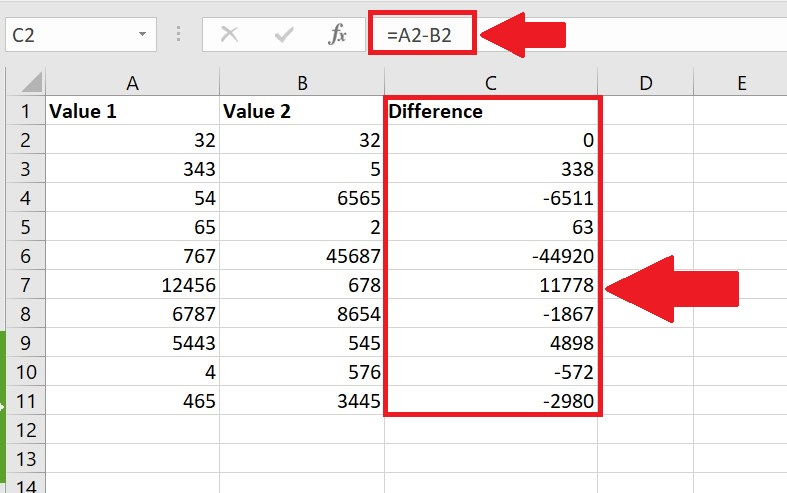# How to use difference formula in excel

In this tutorial, we will learn how to find the difference between two complex numeric values. We will use the subtraction method to find the difference. It subtracts the bigger value from the smaller one. If the user wants to find a difference from smaller value to bigger one, the subtraction symbol will be attached with the answer.  A symbol is used for this, is given below;

Below is the step to find the difference;Excel has a built-in list of special functions that can be used for complex mathematical calculations. In our day to day routine we have to face a situation many times to find the difference between two numeric values, which are usually not easy to solve by mind. Excel supports this scenario, by its built-in function.

### Step 1 – Select the data set cell– To calculate the difference between two numbers by inputting a formula in a new cell. In this case C3 is the cell.
– In this cell, use =A2 – B2.
– As soon as you press the enter key, the result will be displayed.
– Drag it down till the last range, all differences will be calculated.

Picture is given above as an example;

Resultantly, the difference between the value 1 and value 2 has been calculated in the difference column. The values smaller in value 1 column have a difference () symbol attached with answer. Formula is highlighted in the formula cell.